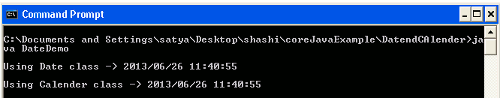Tutorials

# Get current Date and Time

In this example program we have to get the current date at present now. We have used Calendar class and Date class for getting the current date and time instance.

In this example program we have to get the current date at present now. We have used Calendar class and Date class for getting the current date and time instance.

## Get current Date and Time

In this section we will discuss about how to get current date and time in java. Java provide two classes, Date and calendar which will display the current date and time in java. SimpleDateFormat is used  for formatting the date an time. Java provide Date class which is in java.util package. Date class has two constructor they are as follows:

• Date() : This constructor initialize the object with current date and time.
• Date(long ms) : This constructor accept one argument in number of millisecond.

And, some of the method of Date class are as follows :

• after() : Returns true,  if the Date object contain date that is later than the date specified by Date, otherwise return false.
• before() : Returns true, if the Date object contain date that is before than the date specified by Date, otherwise return false.
• getTime() : Returns the number of millisecond from the January 1,1970.
• String ToString() : Convert the date into String.
• equals(Object date) : Returns true if the Date object contain same date and time by the specified date, otherwise return false.

Java provide one more class Calender to get date and time. Both of the class declared as follows :

Using Date Class

```DateFormat dateformat = new SimpleDateFormat("yyyy/MM/dd HH:mm:ss");
Date date = new Date();
dateformat.format(date);```

Using Calender class

```DateFormat dateformat = new SimpleDateFormat("yyyy/MM/dd HH:mm:ss");
Calender cal = new Calender.getInstance();
dateformat.format(cal.getTime());
```

Example : Using the Date and calender class we can get the date and time in java. Using the example we are trying to explain the concept. So, first will create the Dateformat class object for formatting of date and time according to user. After that creating a object of Date class, and similarly creating object for calender class,  using getTime() we can get the time. Now here is the example to show you how to  write the code:

```import java.text.DateFormat;
import java.text.SimpleDateFormat;
import java.util.Calendar;
import java.util.Date;

public class DateDemo {
public static void main(String args[])
{
DateFormat dateformat = new SimpleDateFormat("yyyy/MM/dd hh:mm:ss");
Date date=new Date();
System.out.println("");
System.out.println("Using Date class -> " +dateformat.format(date));

DateFormat dateformat1 = new SimpleDateFormat("yyyy/MM/dd hh:mm:ss");
Calendar cal = Calendar.getInstance();
System.out.println("");
System.out.println("Using Calender class -> " +dateformat1.format(cal.getTime()));

}
}```

Output from the program :